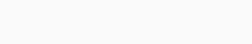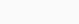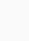## Decimal Arithmetic

#### by FSTC Published on: 8th September 2005

No votes so far! Be the first to rate this post.

The introduction of Arabic numbers into Europe involved more than a way of writing numbers down. It also involved new ways of calculating with pen and paper that were faster and easier and the system of decimal fractions which allowed a simple way of ever more accurate calculations essential for the progress of science.The widely recognised revolution brought about through the use of Arabic numbers replacing the cumbersome Roman numbers involved more than a way of writing the numbers down. It also involved new ways of calculating with pen and paper that were faster and easier and the system of decimal fractions which allowed a simple way of ever more accurate calculations essential for the progress of science. Here we look at some of this contribution.

Part of the Arab approach to mathematics which was undoubtedly helpful in earlier years was the existence of a creative tension between the ‘algebra people’ and the ‘geometry people’. The best examples as evidence are Al-Khwarizmi and Thabit Ibn Qurra respectively. It has been indicated that if the Arab mathematical creativity had been followed earlier, then the period of darkness of some medieval European mathematics could have been avoided. Arabic mathematics quite often appears in numerous studies such as a number of findings, theorems or propositions remarkable for their depth and potential.

Al-Khawarizmi’s arithmetic was the first systematic treatment of arithmetical operations. Al-Khwarizmi discussed the place-value system and rules for performing the four arithmetical operations (addition, subtraction, multiplication and division). Ibn Labban and Al-Uqlidisi presented computational schemes for carrying out operations, such as long multiplication, which were reproduced in medieval Latin texts. Al-Kashi’s The Calculator’s Key presented a comprehensive treatment of arithmetical methods, including operations with decimal fractions.

It has been found that the way the Arabs carried out the basic operations was not very different from the way we use today.

The ‘Sieve’ or ‘Lattice’ Method for Long Multiplication

The Arabs popularised a method for long multiplication known as the ‘Sieve’ or ‘Lattice’ method, which is of historical significance. It has been proved that, even today, the ‘Lattice’ provides a useful diversion in learning long multiplication.

Here the ‘Lattice’ method is illustrated in a very simple case by using it to multiply 2 by 74:This ‘Lattice’ or ‘Sieve’ multiplication shows how Karaji (successor of Al-Khwarizmi) contributed to arithmetic. This multiplication procedure can be explained to a student as follows:

1- the multiplicand is arranged as the horizontal heading in the tableau;

2- the multiplier is arranged vertically in the extreme right column;

3- the products of respective single digits are the decomposed in terms of tens and units within subdivided rectangles arranged horizontally;

4- the product of the multiplication is then performed by the addition in the diagonal channels of operation, starting with diagonal channel on the right representing ones, progressively through channels further left representing tens hundreds, thousands etc. There is space in the diagonal channels to allow for “carrying over” as necessary, and the results are written at the bottom.

Here is a longer multiplication to illustrate the technique:This technique is actually easier than the technique generally taught currently in schools. It involves much less movement of the eyes and it is obvious to see where you are in the calculation at any time and there are less carrying operations. It can also be verified far more easily. Here, for comparison, is the usual technique taught in schools for the same calculation:A good, general survey of the whole area of mathematics is indicative of how rapidly the history of Arab mathematics developed and that this account must now be supplemented in several areas, one of them being the treatment of fractional numbers in Arab mathematics.

It has been stated that neither the Babylonians nor the Chinese had a device or symbol for separating integers from fractions. That symbol is the decimal fraction, which is the invention of Arabs and is credited to them.

Decimal fractions, however, are part of just one of the arithmetic systems used within Islamic science where there were at least three different kinds of arithmetic: the base-60 system of astronomers, the decimal arithmetic that originated in India, and the finger arithmetic of the treasury officials.

It has been mentioned that decimal fractions made their first appearance in Arab mathematics in the Book of Chapters on Indian Arithmetic, written in Damascus in the year 952 or 953 by Abul Hassan Al-Uqlidisi. Al-Uqlidisi considered the problem of successively halving 19 five times, and gave the answer as . The vertical mark on 0 indicates that the decimal fraction part of the number starts with the digit to the right. This notation is perfectly general, so that what in England we write as 0.059375 or in other countries such as Germany 0,059375 would be denoted by . There is evidence that Al-Uqlidisi was aware of the method of multiplying decimal fractions by whole numbers. Al-Samawal (1172) considered decimal fractions in problems of division and extraction of roots. Al-Kashi, astronomer royal for the Timurid Ulugh Beg at Samarkand, used decimal fractions with facility, and several of his remarkable feats of calculations were carried out in both decimal and sexagesimal systems. In the fifteenth century, Al-Kashi provided a comprehensive and systematic treatment of arithmetic operations with decimal fractions. He used different colours or numerical superscript giving the number of decimal places specified: thus  would indicate the decimal fraction 0.35. Therefore, Al-Kashi’s multiplication procedure is identical to the one we use today. The Dutch mathematician Simon Stevin (1548-1620) learnt of the Arab methods of treating decimal fractions. In place of the short vertical line over the last digit of the integer part of the number that was the original notation of Al-Uqlidisi, Stevin use Zero (Sifr in Arabic), so that the number 6.8145 would be represented as . However, it must be mentioned that the present convention of using a decimal point to separate the integer and fractional parts is owed to John Napier.

The Decimal Fraction in Al-Karaji’s School: Al-Samawal

In this part we consider Arabic mathematics in the tenth and twelfth centuries and for the time being we limit ourselves to Alsamawal’s (one of Al-Karaji’s scholars) work. In his Treatise of 1172, Al-Samawal established a usage of decimal fractions. In his work on decimal fractions, Al-Samawal established a rule for approaching the irrational, square and cubic roots; a rule that is called the “rule of zero”. The general formulation of this rule is to be found in Al-Samawal’s Treatise isObviously, the approximation obtained according to this rule includes a decimal fraction. This sporadic usage of decimal fractions according to Al-Samawal’s theoretical exposition came at the end of his working following an exposition of methods and problems of approximation.

One of Al-Samawal’s aims was to unify and generalise. That he aimed to have “indefinite correction of fractions”. He meant to give the fractions a form so that they can be calculated like integers, and from then on, it is possible to correct the approximation of different operations indefinitely. Having reached this stage in his exposition of decimal fractions, Al-Samawal was quite naturally confronted with the famous problem of notation, and consequently induced to deal with it indirectly at least. However, this notation had to fulfil two requirements whether expressed in words or symbolically. The first one, theoretical, was partially fulfilled by expressing polynomials by means of tables: the limited or unlimited representation of any real number then known had to be possible; the second one, of a practical nature, concerns the possibility of pronouncing such a presentation. Satisfying the latter condition is dependent on the integration of the body of decimal fractions into a practice other than purely bookish. In such a context the problem of the notation of decimal fractions had therefore to be posed in the tradition of the tabular method. Moreover, Al-Samawal gave examples that tend to confirm this method. He applied the same operations he effected on integers written as decimals to decimal fractions without justification.

As an example showing the tabular method, is the simple division of 210 by 13. Al-Samawal emphasized in the first place that this division may be continued as far as one wants; that if we continue the operation to the places we want, and if we restrict ourselves to five places, we obtain the result written as follows:

 16 1 5 3 8 4 integers Part of ten Parts of a hundred Parts of a thousand Parts of ten thousand Parts of one hundred thousand

As we can see this notation is based on the principle of separating the integer part and represent the fractional part according to Al-Samawal’s technique. But this notation was difficult to pronounce and consequently restricted in practical scope.

Similarly, in other examples, Al-Samawal modified it in the sense just indicated: this corrections emphasize the succession of places rather than terms: parts of ten, parts of a hundred, parts of thousand, etc. and make pronunciation possible. It is obvious, that there is an improvement in Al-Samawal’s second example, the extraction of the square root of 10, where he writes down the result as follows:

 Tens Units Tenths Tenths Tenths Tenths Tenths Tenths of tenth of a tenth of a tenth of a tenth of a tenth of a tenth of a tenth of a tenth of a tenth of a tenth of a tenth of a tenth of a tenth of a tenth of a tenth 3 1 6 2 2 7 7

Here, one can find that, although the notational principle is fundamentally identical to the previous one, Al-Samawal clearly wanted to bring out the succession of places as well as their rank, by representing the same term as often as necessary. Therefore, instead of using the expression “parts of ten, parts of a hundred, parts of a thousand, etc.” the equivalent can be written as following:The nth place is thus indicated by the repetition of the term n times, the “tenth”-(ushr in Arabic).

This unification assumes an importance that may surprise a modern reader; in fact this importance lies at the origin of a proper noun which was used later to name these fractions, “ushria”, or “ashria”, i.e. tenths. We can say that despite this improvement in notation, the difficulty remains if one wants to pronounce such a number. To overcome this difficulty, Al-Samawal was inspired by a notation then used for ordinary fractions and relates the fractional part to the same denominator. He thus achieved the following notation,

 3 1 6 2 2 7 7 1 0 0 0 0 0 0

Which reads: 3 units plus 162277 of 1.000.000, or as Al-Samawal wrote:

If we want all the fractions obtained to have the same denominator, we transfer the place of the units as well as (the places that following it-tenths, hundreds and the other (places) of integers to a higher line and underneath the other places (of the fraction) we write down zeros, and after the zeros, one.

To conclude his exposition, Al-Samawal briefly recalled the initial purpose of the theory of decimal fractions: to enable the application of various operations such as division and the extraction of nth root of fractions in the same way as for integers and consequently made the indefinite correction of approximations more obvious and easier.

Al-Samawal’s essential idea was that: the nth non-decimal root of any positive number is the limit of an ascending sequenceof decimal values, being an approached valueto the nearest decimal point.

Al-Samawal concluded, “we operate to determine the side of a cube, of a square-square, a square-cube and another [powers] this method enables us to understand fully the exact operations for partition and obtain an infinite number of answers, each one being more precise and closer to the truth than the preceding one”.

It must be said that, with Al-Samawal, the theory of decimal fractions emerged within the context of the problem of the extraction of the nth root of a number and problems of approximation. Thus, Al-Kaaji’s school was the core of the first exposition of the decimal fractions approach.

References

1- F.J. Swetz: From Five Fingers to Infinity; Open Court; Chicago; 1994; pp. 289-92.

2- G.G. Joseph: The Crest of the Peacock; Penguin Books; 1991.

3- J.L. Berggren: History of Mathematics in The Islamic World: The Present State of The Art. Middle East Studies Association Bulletin 19 (1985), pp. 9-33.

4- R. Rashed: The Development of Arabic Mathematics: between arithmetic and algebra. Dordrecht: Kluwer. 1994.

No votes so far! Be the first to rate this post.

## FROM THIS AUTHOR#### 1001 Inventions vs. 1001 nights

by FSTC#### Ahmad Ibn Fadhlan in Northern Europe: A Survey of his Account of Russian Vikings in the 10th Century

by FSTC#### Dr. Farouk El-Baz receives 1001inventions book

by FSTC#### Mshatta Palace, Jordan; 743-744 CE

by FSTCby FSTC#### Ribat of Soussa, Muslim invention of rib vaulting?

by FSTC#### Cordoba, European Jewel of the Middle Ages

by FSTC#### Kutubiya Mosque (1158)

by FSTC#### FSTC Activity Report 2015

by FSTC#### Al-Qarawiyyin Mosque and University

by FSTC#### Al-Qayrawan Mosque

by FSTC#### Presentation on The Muslims’ Great Contributions

by FSTC#### FSTC Renews Scientific Contacts with Aleppo University

by FSTC#### Precious Records of Eclipses in Muslim Astronomy and History

by FSTC#### Architecture of Muslim Spain and North Africa

by FSTC#### Pharmacology in the Making

by FSTC#### Muslim Heritage in the World Conference on Intellectual Capital

by FSTC#### Al-Hakim Mosque, Cairo (990-1012)

by FSTC#### Illustrious Names in the Heavens: Arabic and Islamic Names of the Moon Craters

by FSTC#### Ibn Battuta and the 14th Century Muslim World

by FSTCby FSTC